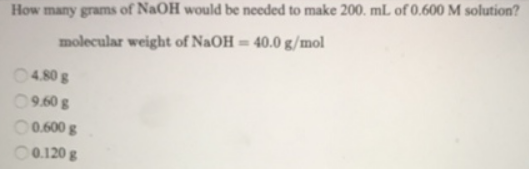# Problem: How many grams of NaOH would be needed to make 200. mL of 0.600 M solution? molecular weight of NaOH = 40.0 g/mol

###### FREE Expert Solution
92% (295 ratings)###### Problem Details

How many grams of NaOH would be needed to make 200. mL of 0.600 M solution?

molecular weight of NaOH = 40.0 g/molFrequently Asked Questions

What scientific concept do you need to know in order to solve this problem?

Our tutors have indicated that to solve this problem you will need to apply the Molarity concept. You can view video lessons to learn Molarity. Or if you need more Molarity practice, you can also practice Molarity practice problems.